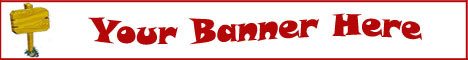# What's Up East Coast ColumnYou got the lowdown in the column, now get the breakdown. (A very special THANK YOU to Diane Pullen from WUEC for making this column possible!)

ADVERTISE in the popular WUEC magazine and post your events for free! This full colour booklet has been around for over 5 years and several thousands of hard copies are printed and distributed bi-monthly in the Eastern Cape area.
Contact me TODAY to get your listing up. It costs less than you think!

26 Oct 2015, 11:19 am

## Not so Average

This week I had to calculate the averages of test results for an important report. Students generally did really well, mostly in the high 70’s and 80’s. Yet the averages were very low due to a few students who did not write the tests. Results for these students were therefore automatically entered as “0” and negatively affected the averages of the whole group.

As I could not completely remove these students from the lists, I needed a way to calculate the averages while IGNORING any ZERO values.

So let’s look at this an old favourite in Excel: the AVERAGE formula. (Remember that older versions of Excel still require the use of commas instead of semi-colons!)

• NUMBERS IN A CONTIGUOUS ROW OR COLUMN

A simple AVERAGE formula might look something like this:

=AVERAGE(A1:A9)

This will calculate an average of ALL the numbers in the range A1:A9, including zero values.

• NUMBERS NOT IN A CONTIGUOUS ROW OR COLUMN

Should you want to calculate an average of only certain values, the formula might look something like this:

=AVERAGE(A2:A4;A7)

In this example Excel averages the top three and the last number in the range A2:A7

• IGNORING ZERO (0) VALUES

To ignore zero values, combine the AVERAGE formula with the IF formula. Here is an example:

=AVERAGE(IF(A2:A7<>0;A2:A7;""))

In this example Excel averages the numbers in the list except those that contain zero.

Note: The formula must be entered as an array formula. After typing the formula into the cell, select the cell, press F2, and then press CTRL+SHIFT+ENTER. If the formula is not entered as an array formula, the error #VALUE! is returned.

The average is also called the mean.

I hope you enjoyed this mini course. To book a private training session contact me on tiamari@tiamari.com

Happy clicking!
Tiamari

Showing all records: